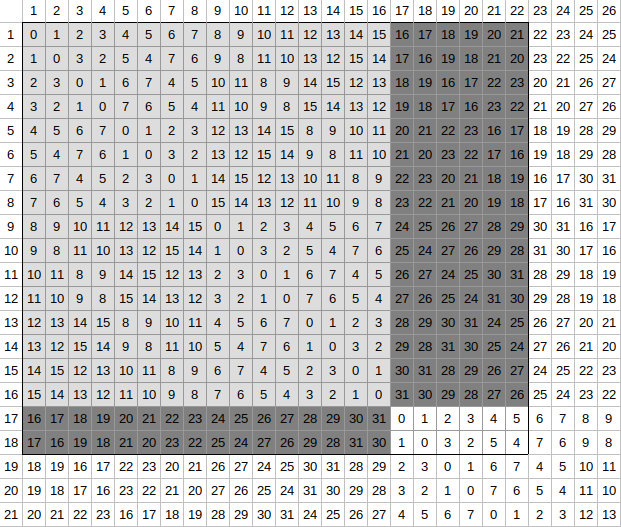Become Immortal is at this point the only 1kyu kata I tried (and solved) on CodeWars. It took me 3-4 days to solve it. It was definitely pretty damn difficult to figure out, but in the end I managed it myself.

Looking at the example tests it was immediately obvious that trying to generate the actual rectangles and xoring everything was out of question. For the heck of it I tried once, but even for the last “basic test” I’d run out of memory (on my desktop) very quick. Sure it works fine if the rectangle is 30-40 rows and columns, but not for scales in the millions. I used it to dump rectangles on the console and double check my solution.

First step, dump to console. Looking at the numbers it’s soon clear that all numbers are there in every square. However, my first solution based on that assumption kept crapping itself, and making the table a bit bigger I realized that there are “breaking points” and weird fractal-like patterns. I tried coloring the numbers on the console, and the patterns became even more obvious. Turns out there’s a name for it too: munching squares.

The problem is that in the kata I’m given a rectangle which is not necessarily a square, but I only know the rule (that all numbers are in every row and column, just like in sudoku or magic squares) for squares sized a power of two (2, 4, 8 and so on). What do I do with the rest? Recursion.First, I make the rectangle horizontal (eg swap the sides so that the width will be bigger). It works because the pattern is symmetric and it makes it easier to write conditionals and such. Next I find the largest power of two smaller than or equal to the width (using logarithm).

The easiest solution is when the width is exactly a power of two, because then there are no leftovers (remember, I made the width the bigger number). Then all numbers are present in every row, and I just need to multiply that by the number of rows.

When there are leftovers though, it’s a bit more complicated. One side of the leftover on the right and the bottom (dark gray above) is a power of 2 (the size of the light gray square I fit in), so those are again the case I described above. For the fourth piece though (white above), I need to use recursion.

Calculate the same for that small rectangle (with sides that are not necessarily sized a power of two), and keep repeating until there’s none left. I was a bit scared of going too deep in the stack with very big numbers, but that wasn’t so much of an issue with the exponential nature of the squares.

Shifting all values because of the “lag” in the kata was pretty annoying, but at least it’s simple to test and experiment with, because it works the same for small numbers. Just figure out how many zeroes there are in a row (and in a rectangle with a side a power of two there will be the same number of zeroes in every row). Then it’s the sum of all integers in a range.

I had some trouble figuring out the number of zeroes, and the “offset” I needed to use as the starting point for the individual rectangles, but again dumping lots of (relatively small) tables and testing in the console or (like in the screenshot above) visualizing in Calc or Excel or whatever helps a lot.

My solution clocked at around 4 seconds for all the 900 tests (which is definitely better than eating 6 gigs of memory instantly), which could still use some improvement. The way I used modulo (just on the end result) could probably be optimized and there may be shortcuts or laws I could abuse to calculate the sums even faster. At this point however, I can’t really come up with anything better. Maybe sometime later.